# Linear Inequalities and Absolute Value Inequalities 1 Express

• Slides: 16Linear Inequalities and Absolute Value Inequalities1. Express each interval in set-builder notation and graph the interval on a number line: (Similar to p. 185 #4)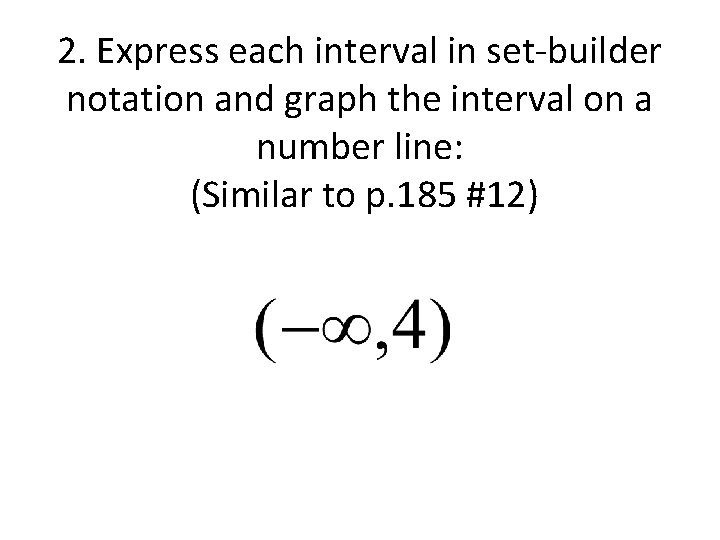2. Express each interval in set-builder notation and graph the interval on a number line: (Similar to p. 185 #12)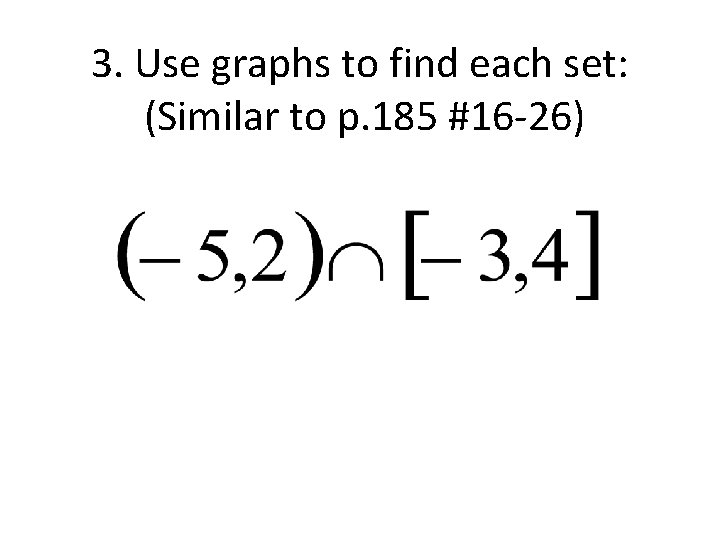3. Use graphs to find each set: (Similar to p. 185 #16 -26)4. Use graphs to find each set: (Similar to p. 185 #16 -26)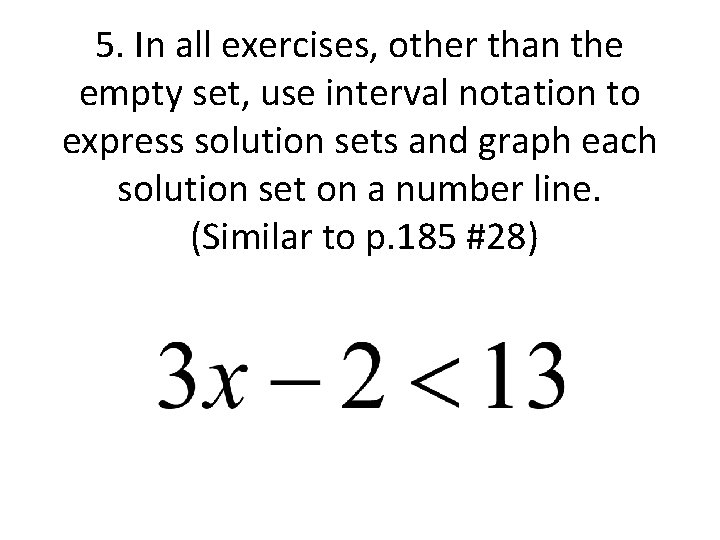5. In all exercises, other than the empty set, use interval notation to express solution sets and graph each solution set on a number line. (Similar to p. 185 #28)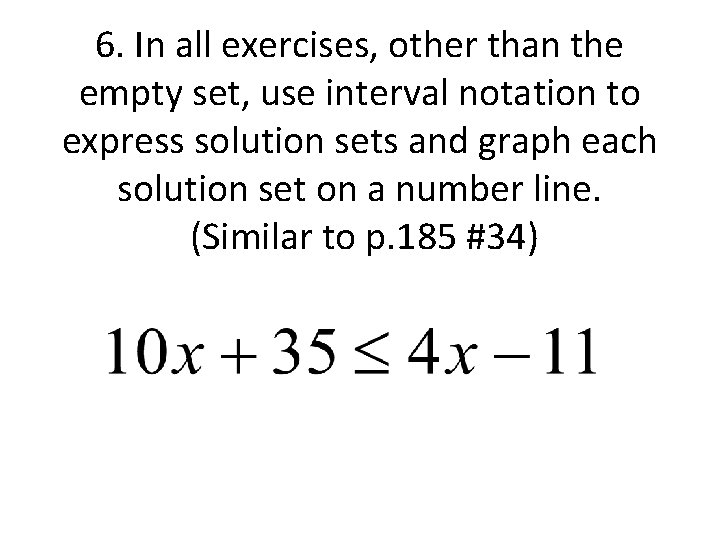6. In all exercises, other than the empty set, use interval notation to express solution sets and graph each solution set on a number line. (Similar to p. 185 #34)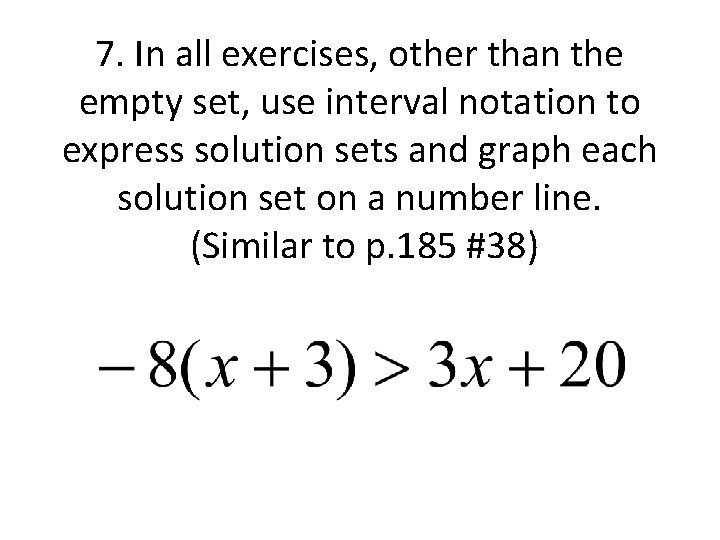7. In all exercises, other than the empty set, use interval notation to express solution sets and graph each solution set on a number line. (Similar to p. 185 #38)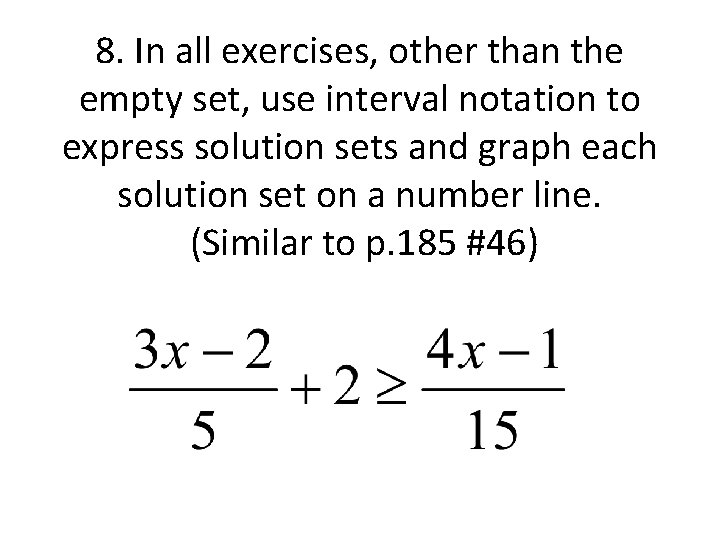8. In all exercises, other than the empty set, use interval notation to express solution sets and graph each solution set on a number line. (Similar to p. 185 #46)9. Solve each compound inequality: (Similar to p. 185 #56)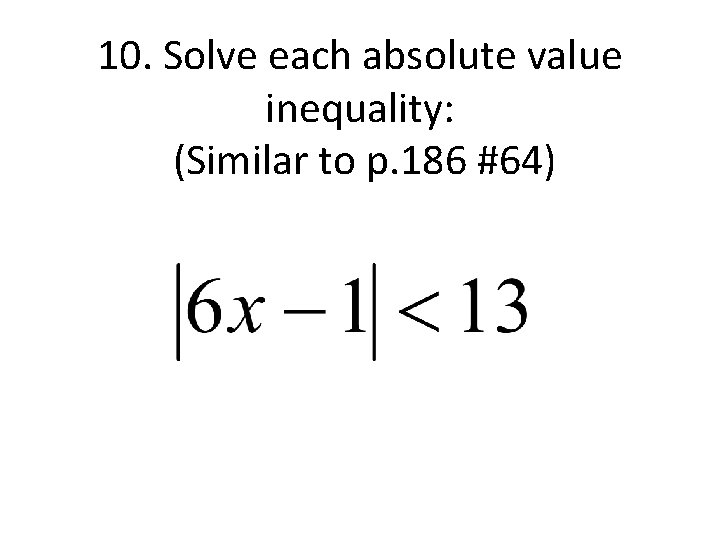10. Solve each absolute value inequality: (Similar to p. 186 #64)11. Solve each absolute value inequality: (Similar to p. 186 #74)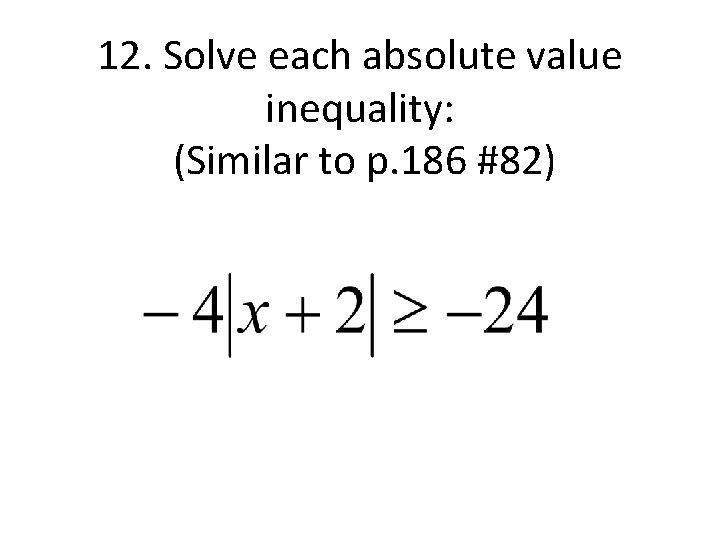12. Solve each absolute value inequality: (Similar to p. 186 #82)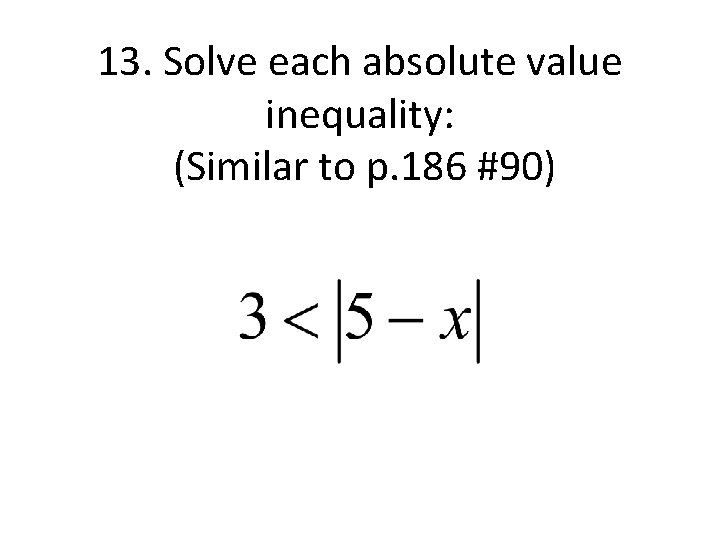13. Solve each absolute value inequality: (Similar to p. 186 #90)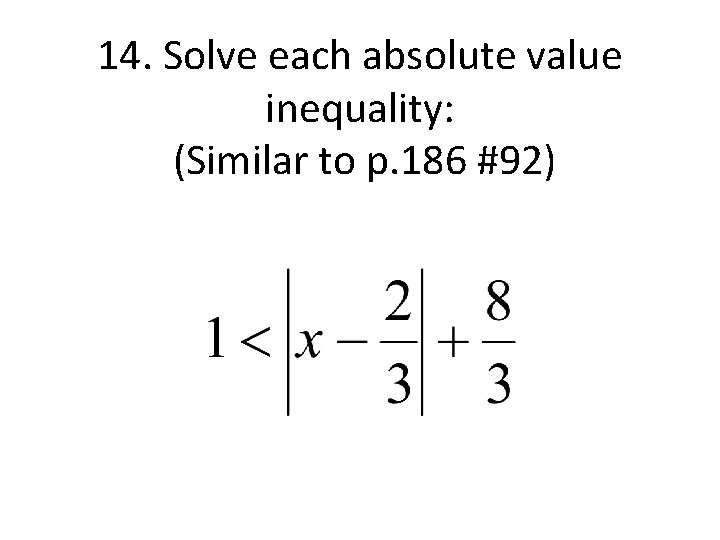14. Solve each absolute value inequality: (Similar to p. 186 #92)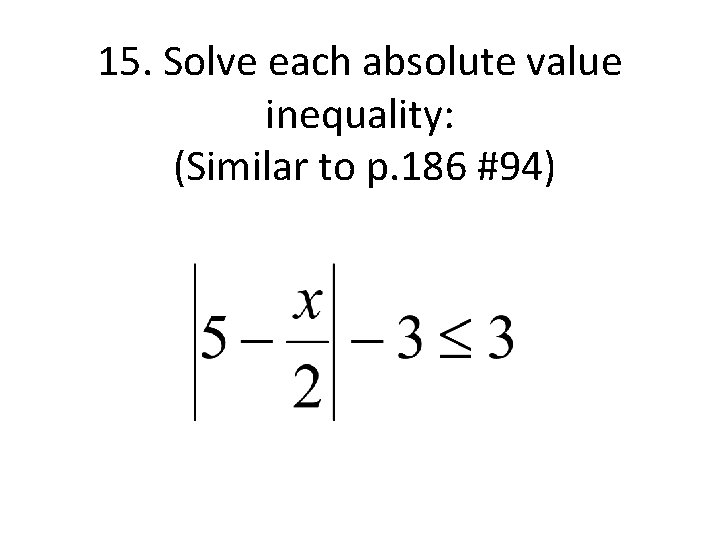15. Solve each absolute value inequality: (Similar to p. 186 #94)